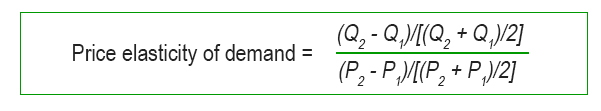# How to Calculate Price Elasticities Using the Midpoint Formula

| Published Nov 30, 2018

Price elasticity of demand is a measure that shows how much quantity demanded changes in response to a change in price.  It is calculated as the percentage change in quantity demanded divided by the percentage change in price (see also Elasticitiy of Demand). However, as you will notice sooner or later, this formula has an annoying limitation: It will not produce distinct results when we use it to calculate the price elasticity of two different points on a demand curve.

Fortunately, there is a simple trick we can use to avoid this issue: the so-called midpoint method to calculate price elasticities. In the following paragraphs we will learn step-by-step how to use the midpoint formula to claculate price elasticities. But before we do that, let’s take a step back and look at why the problem we mentioned above arises in the first place.

## Elasticity Between Two Points on a Curve

When we try to calculate the price elasticity of demand between two points on a demand curve as described above, we quickly see that the elasticity from point A to point B seems different from the elasticity from point B to point A. While this seems odd at first, it actually makes perfect sense, because we generally calculate percentage changes relative to their initial value. Now if we move from point A to point B, the initial value is at level A. However, if we move from point B to point A, the initial value is at level B. To illustrate this, let’s look at the graph below.As you can see, at point A, the pice is USD 2.00 and quantity is 100 units. Meanwhile, at point B price and quantity are USD 3.00 and 80 units respectively. That means, going from point A to point B, the price increases by 50% (i.e. [3-2]/2) while quantity decreases by 20% (i.e. [80-100]/100). This indicates a price elasticity of 0.4 (i.e. 20/50). By contrast, going from point B to point A, the price only decreases by 33% (i.e. [2-3]/3) while quantity increases by 25% ([100-80]/80). This indicates a price elasticity of 0.75 (i.e. 25/33).

## The Midpoint Formula

As mentioned before, we can avoid this problem by using the so-called midpoint method. Usually when we calculate percentage changes we divide the change by the initial value and multiply the result by 100. Unlike that, the midpoint formula divides the change by the average value (i.e. the midpoint) of the initial and final value.

In the case of our example (see above) the average price is USD 2.50 (i.e. [2.00 + 3.00]/2) and the average quantity demanded is 90 (i.e. [100 + 80]/2). Thus, according to the midpoint method, a change from point A to point B (i.e. USD 2.00 to 3.00) is considered a 40% increase (i.e. [3.00 – 2.00]/2.50). Similarly, a change from point B to point A (i.e. USD 3.00 to 2.00) is considered a 40% decrease (i.e. [2.00 – 3.00]/2.50).

As we can see, the percentage change is the same regardless of the direction we move. Of course, this also holds true for the quantity demanded. A move from point A to point B (i.e. 100 to 80) is considered a 22% decrease (i.e. [80 – 100]/90). Similarly, a move from point B to point A (i.e. 80 to 100) is considered a 22% increase ([100 – 80]/90).

With the percentage changes calculated with the midpoint method, we can now compute a distinct price elasticity of demand beteween points A and B. To do this, we use the following formula:The formula actually looks a lot more complicated than it really is. As a matter of fact, all we need to do at this point is divide the percentage change in quantity demanded we calculate above by the percentage change in price. As a result, the price elasticity of demand equals 0.55 (i.e. 22/40).

Please note: Unless stated otherwise, is advisable to use the midpoint method whenever you have to calculate percentage changes and price elasticities between two points on a curve.

## In a Nutshell

Price elasticity of demand shows how much quantity demanded changes in response to a change in price. It is calculated as the percentage change in quantity demanded divided by the percentage change in price. However, this approach does not produce distinct results when we use it to calculate the price elasticity of two different points on a demand curve (i.e. results are different based on the direction of change). The midpoint formula computes percentage changes by dividing the change by the average value (i.e. the midpoint) of the initial and final value. As a result, it produces the same result regardless of the direction of change. Therefore is advisable to use the midpoint method whenever you have to calculate percentage changes and price elasticities between two points on a curve.